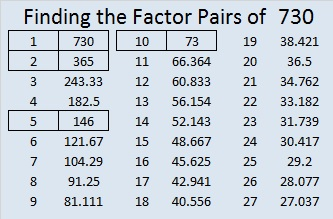# 730 and Level 5

Two years have 730 days when neither year is a leap year.

• 730 is a composite number.
• Prime factorization: 730 = 2 x 5 x 73
• The exponents in the prime factorization are 1, 1, and 1. Adding one to each and multiplying we get (1 + 1)(1 + 1)(1 + 1) = 2 x 2 x 2 = 8. Therefore 730 has exactly 8 factors.
• Factors of 730: 1, 2, 5, 10, 73, 146, 365, 730
• Factor pairs: 730 = 1 x 730, 2 x 365, 5 x 146, or 10 x 73
• 730 has no square factors that allow its square root to be simplified. √730 ≈ 27.01851.Here are some statistics that WordPress gave me just before 2015 ended: findthefactors.com 2015annual report.Print the puzzles or type the solution on this excel file: 12 Factors 2015-12-28

———————————————————

Here a few more facts about the number 730:

730 is the sum of consecutive numbers several ways:

• 181 + 182 + 183 + 184 = 730; that’s 4 consecutive numbers.
• 144 + 145 + 146 + 147 + 148 = 730; that’s 5 consecutive numbers.
• 27 + 28 + 29 + 30 + 31 + 32 + 33 + 34 + 35 + 36 + 37 + 38 + 39 + 40 + 41 + 42 + 43 + 44 + 45 + 46 = 730; that’s 20 consecutive numbers.

730 is also the sum of two squares two ways:

• 27² + 1² = 730
• 21² + 17² = 730

Because 5 and 73 are two of its factors, 730 is the hypotenuse of FOUR Pythagorean triples (none are primitive):

• 54-728-730, calculated from 2(27)(1), 27² – 1², 27² + 1²
• 152-714-730, calculated from 21² – 17², 2(21)(17), 21² + 17²
• 438-584-730, and 146 is their GCF (greatest common factor).
• 480-550-730, and 10 is their GCF.

730 is a palindrome in three bases:

• 1000001 BASE 3
• 1001 BASE 9
• 101 BASE 27

———————————————————This site uses Akismet to reduce spam. Learn how your comment data is processed.